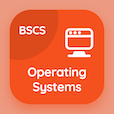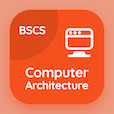Computer Science Courses Online

Digital Logic Design Certification Exam Tests

Digital Logic Design Practice Test 15

# Introduction to Asynchronous Sequential Logic Quiz Answers PDF - 15

The Book Introduction to Asynchronous Sequential Logic Quiz Questions and Answers, introduction to asynchronous sequential logic Quiz MCQs PDF, chapter 2-15 to download online logic design degree courses. Study Asynchronous Sequential Logic MCQ Questions PDF, introduction to asynchronous sequential logic Multiple Choice Questions (MCQ Quiz) for online college degrees. The e-Book Introduction to Asynchronous Sequential Logic Quiz App Download: introduction to asynchronous sequential logic, flip-flops, adder and subtractors, design with multiplexers, rectangular shape symbols test prep for 2 year computer science degree.

The Quiz: Which of the following sequential circuits generate the feedback path due to the cross-coupled connection from the output of one gate to the input of another gate? PDF, "Introduction to Asynchronous Sequential Logic" App (Android & iOS) Free with asynchronous, synchronous, excitation variables, and continuously change choices to learn online certificate courses. Practice asynchronous sequential logic questions and answers, Google eBook to download free sample for computer and information science.

## Logic Design Quiz Online: Introduction to Asynchronous Sequential Logic MCQs - 15

MCQ: Which of the following sequential circuits generate the feedback path due to the cross-coupled connection from the output of one gate to the input of another gate?

A) synchronous
B) asynchronous
C) excitation variables
D) continuously change

MCQ: The sequential circuit is also called

A) combinational circuits
B) sequential circuits
C) logic circuits
D) complex circuits

MCQ: Integrated Circuit (IC) type 7483 consists of

A) 1bit IC
B) 2bits IC
C) 3bits IC
D) 4bits IC

MCQ: What does 1 represent in most of the logic gates?

A) 0V
B) 1V
C) 5V
D) 10V

MCQ: Negation is represented by the sign of

A) small circle
B) large square
C) large triangle
D) small triangle

### Mock Tests: Digital Logic Design Course Prep

Download Digital Logic Design Quiz App, Operating Systems MCQ App, and Computer Architecture MCQs App to install for Android & iOS devices. These Apps include complete analytics of real time attempts with interactive assessments. Download Play Store & App Store Apps & Enjoy 100% functionality with subscriptions!